Lesson 22 AC Power Factor and Power Factor

• Slides: 24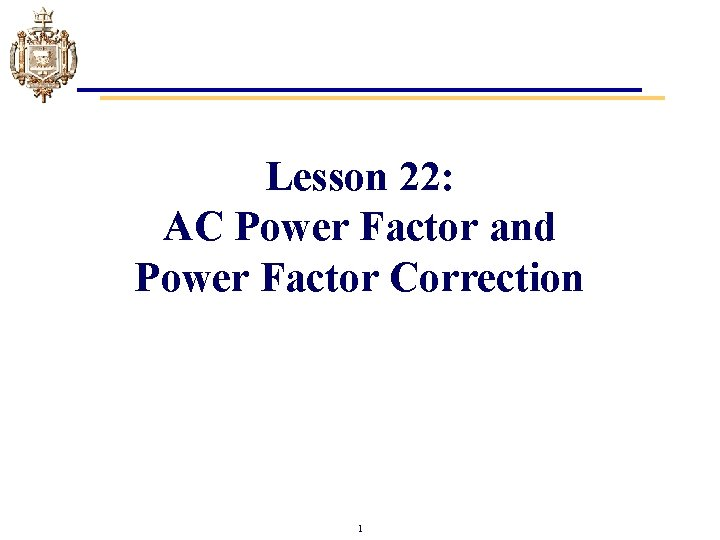Lesson 22: AC Power Factor and Power Factor Correction 1Learning Objectives • Define power factor. • Define unity, leading and lagging power factors. • Define power factor correction and unity power factor correction. • Calculate the inductor or capacitor value required to correct AC series parallel networks to the desired apparent power. • Compare currents, voltages, and power in AC series parallel networks before and after power factor correction. 2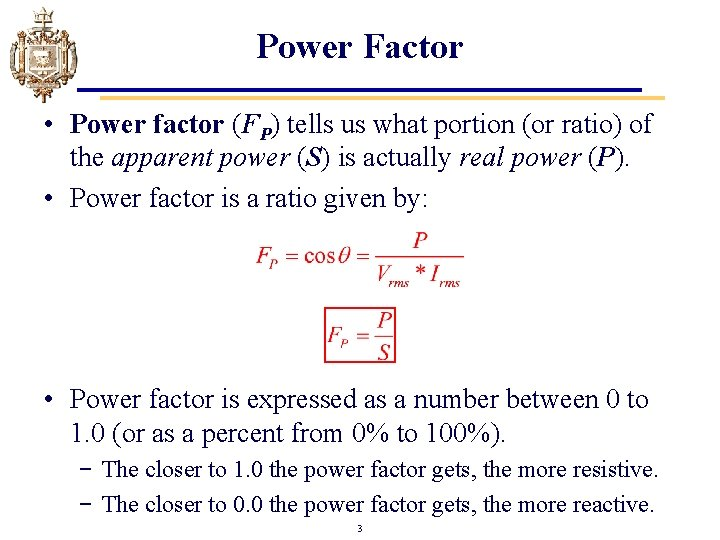Power Factor • Power factor (FP) tells us what portion (or ratio) of the apparent power (S) is actually real power (P). • Power factor is a ratio given by: • Power factor is expressed as a number between 0 to 1. 0 (or as a percent from 0% to 100%). − The closer to 1. 0 the power factor gets, the more resistive. − The closer to 0. 0 the power factor gets, the more reactive. 3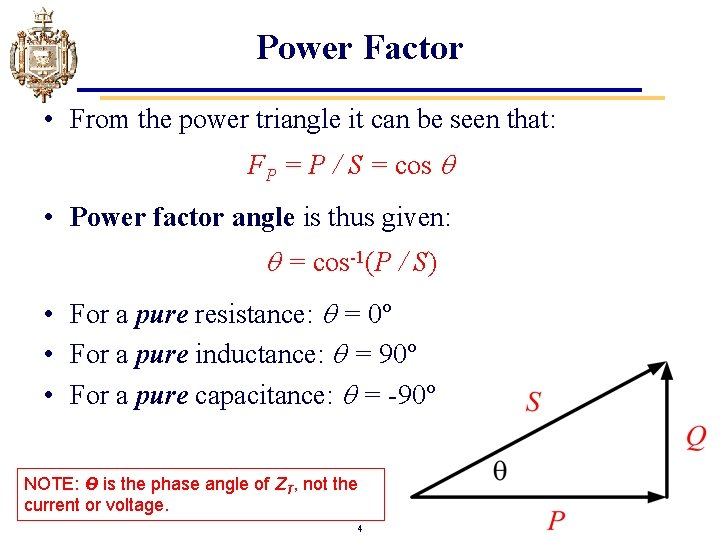Power Factor • From the power triangle it can be seen that: FP = P / S = cos • Power factor angle is thus given: = cos-1(P / S) • For a pure resistance: = 0º • For a pure inductance: = 90º • For a pure capacitance: = -90º NOTE: Ө is the phase angle of ZT, not the current or voltage. 4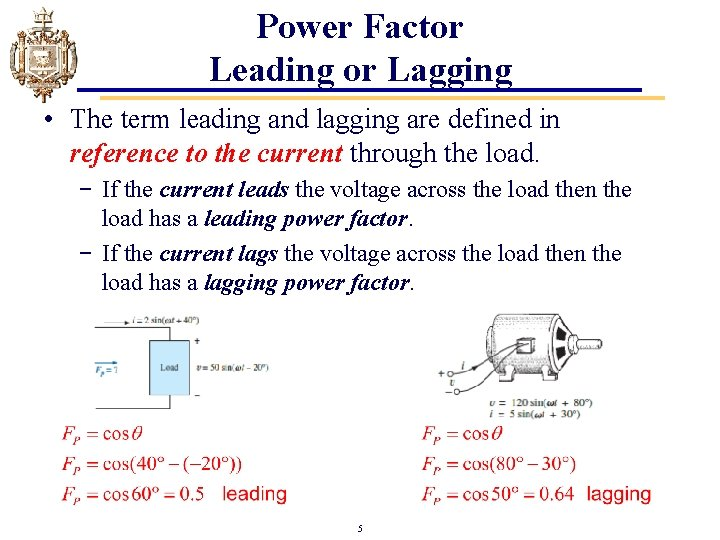Power Factor Leading or Lagging • The term leading and lagging are defined in reference to the current through the load. − If the current leads the voltage across the load then the load has a leading power factor. − If the current lags the voltage across the load then the load has a lagging power factor. 5Unity Power Factor (FP = 1) • Unity Power Factor implies that all of a load’s apparent power is real power (S = P). • If FP = 1, then = 0º. • It could also be said that the load looks purely resistive. • Load current and voltage are in phase. 6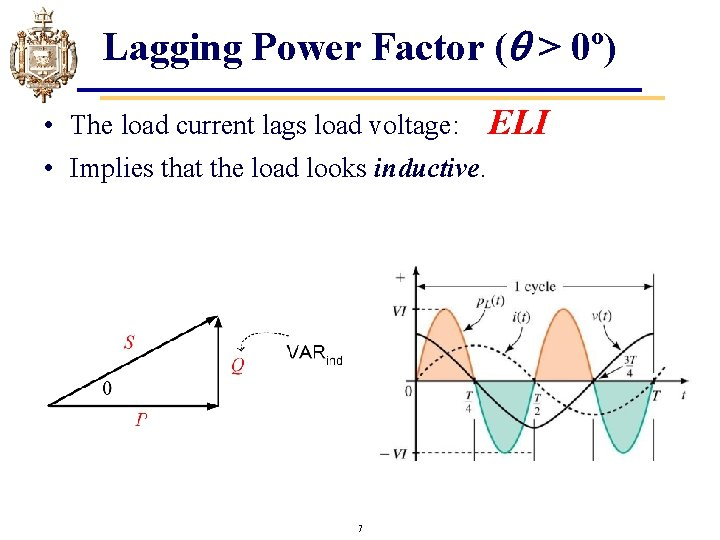Lagging Power Factor ( > 0º) • The load current lags load voltage: ELI • Implies that the load looks inductive. 7Example Problem 1 a. Determine P, Q, S and the FP for this circuit. a. Draw the power triangle. b. Is it a leading or lagging power factor? c. Is the circuit inductive or capacitive? . . In Rectangular: => 53. 4% real power 9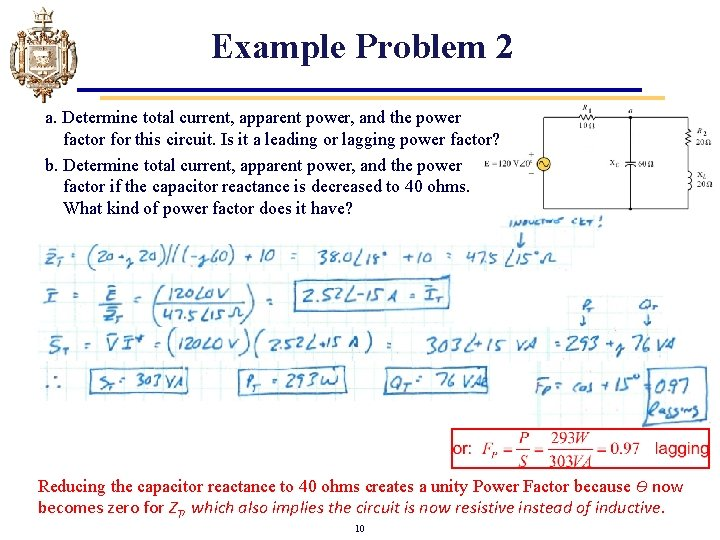Example Problem 2 a. Determine total current, apparent power, and the power factor for this circuit. Is it a leading or lagging power factor? b. Determine total current, apparent power, and the power factor if the capacitor reactance is decreased to 40 ohms. What kind of power factor does it have? Reducing the capacitor reactance to 40 ohms creates a unity Power Factor because Ѳ now becomes zero for ZT, which also implies the circuit is now resistive instead of inductive. 10Why is Power Factor Important? • Consider the following example: A generator is rated at 600 V and supplies one of two possible loads. Load 1: P = 120 k. W, FP = 1 Load 2: P = 120 k. W, FP = 0. 6 • Determining how much current (I) is required is one such reason. . . 11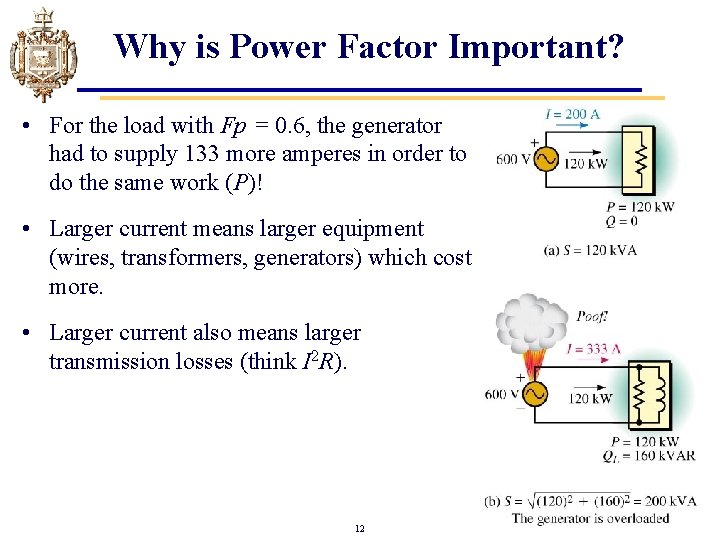Why is Power Factor Important? • For the load with Fp = 0. 6, the generator had to supply 133 more amperes in order to do the same work (P)! • Larger current means larger equipment (wires, transformers, generators) which cost more. • Larger current also means larger transmission losses (think I 2 R). 12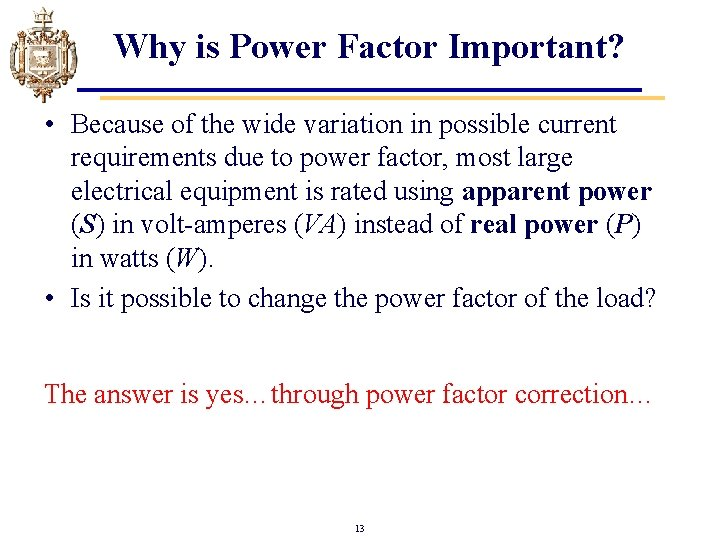Why is Power Factor Important? • Because of the wide variation in possible current requirements due to power factor, most large electrical equipment is rated using apparent power (S) in volt-amperes (VA) instead of real power (P) in watts (W). • Is it possible to change the power factor of the load? The answer is yes…through power factor correction… 13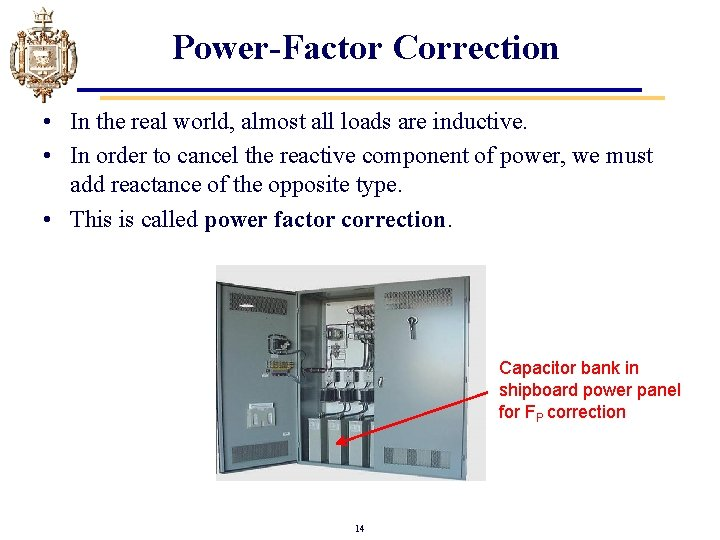Power-Factor Correction • In the real world, almost all loads are inductive. • In order to cancel the reactive component of power, we must add reactance of the opposite type. • This is called power factor correction. Capacitor bank in shipboard power panel for FP correction 14Power-Factor Correction • In practice, almost all loads (commercial, industrial and residential) look inductive (due to motors, fluorescent lamp ballasts, etc. ). • Hence, almost all power factor correction consists of adding capacitance. 15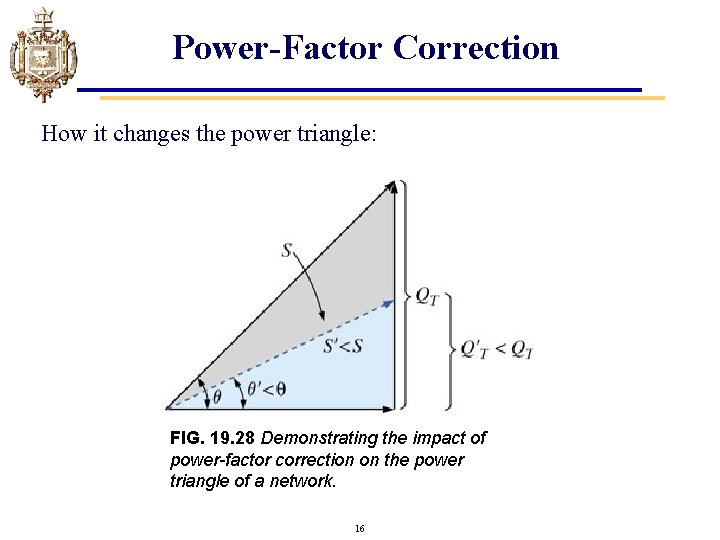Power-Factor Correction How it changes the power triangle: FIG. 19. 28 Demonstrating the impact of power-factor correction on the power triangle of a network. 16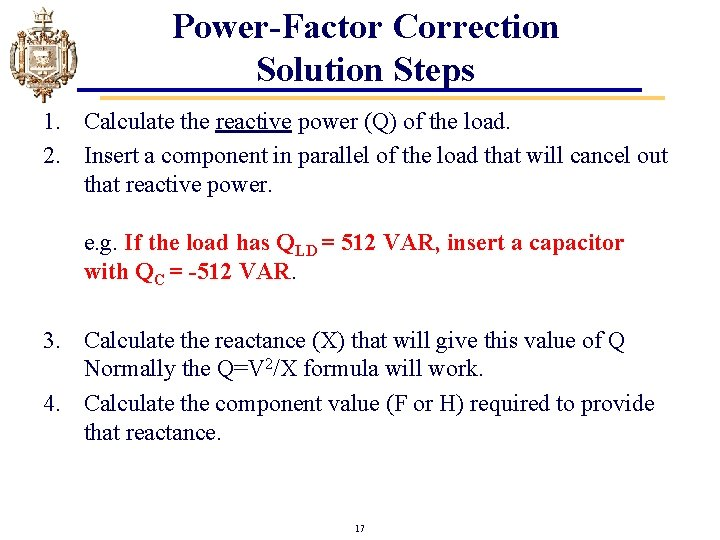Power-Factor Correction Solution Steps 1. Calculate the reactive power (Q) of the load. 2. Insert a component in parallel of the load that will cancel out that reactive power. e. g. If the load has QLD = 512 VAR, insert a capacitor with QC = -512 VAR. 3. Calculate the reactance (X) that will give this value of Q Normally the Q=V 2/X formula will work. 4. Calculate the component value (F or H) required to provide that reactance. 17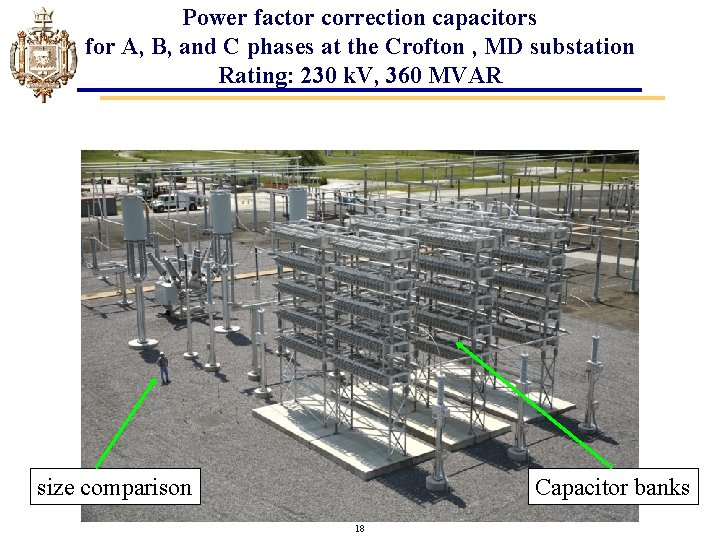Power factor correction capacitors for A, B, and C phases at the Crofton , MD substation Rating: 230 k. V, 360 MVAR size comparison Capacitor banks 18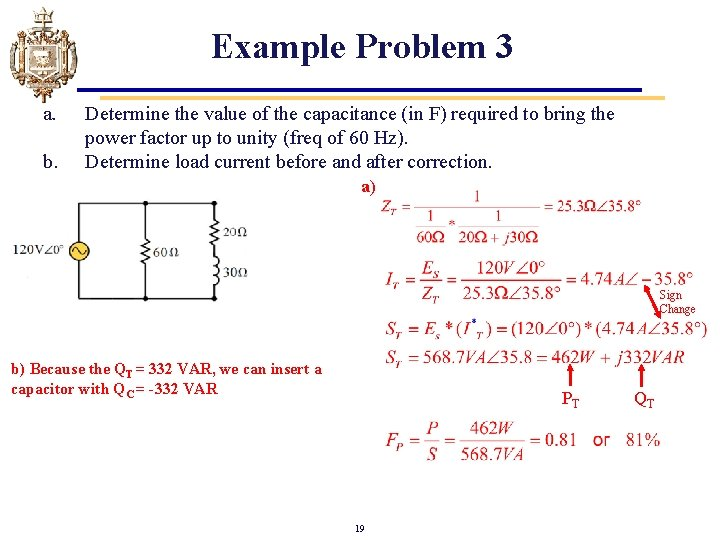Example Problem 3 a. b. Determine the value of the capacitance (in F) required to bring the power factor up to unity (freq of 60 Hz). Determine load current before and after correction. a) Sign Change b) Because the QT = 332 VAR, we can insert a capacitor with QC = -332 VAR PT 19 QT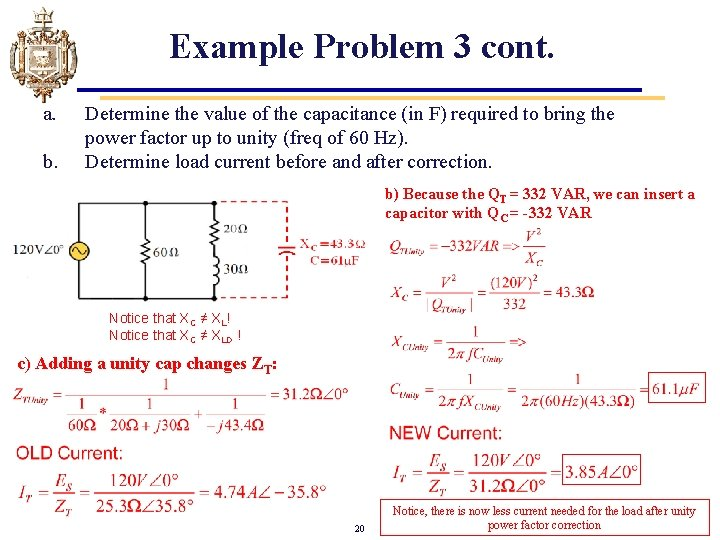Example Problem 3 cont. a. b. Determine the value of the capacitance (in F) required to bring the power factor up to unity (freq of 60 Hz). Determine load current before and after correction. b) Because the QT = 332 VAR, we can insert a capacitor with QC = -332 VAR Notice that XC ≠ XL! Notice that XC ≠ XLD ! c) Adding a unity cap changes ZT: 20 Notice, there is now less current needed for the load after unity power factor correction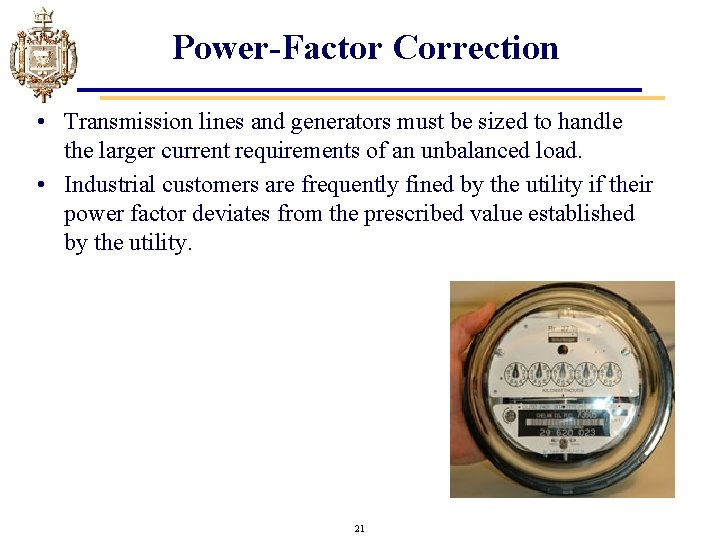Power-Factor Correction • Transmission lines and generators must be sized to handle the larger current requirements of an unbalanced load. • Industrial customers are frequently fined by the utility if their power factor deviates from the prescribed value established by the utility. 21Example Problem 4 a. b. c. Determine S, PT, QT, and FP. Determine the value of the capacitance (in F) required to bring the power factor up to unity (freq of 60 Hz). Determine generator current before and after correction. a) 11738 W 22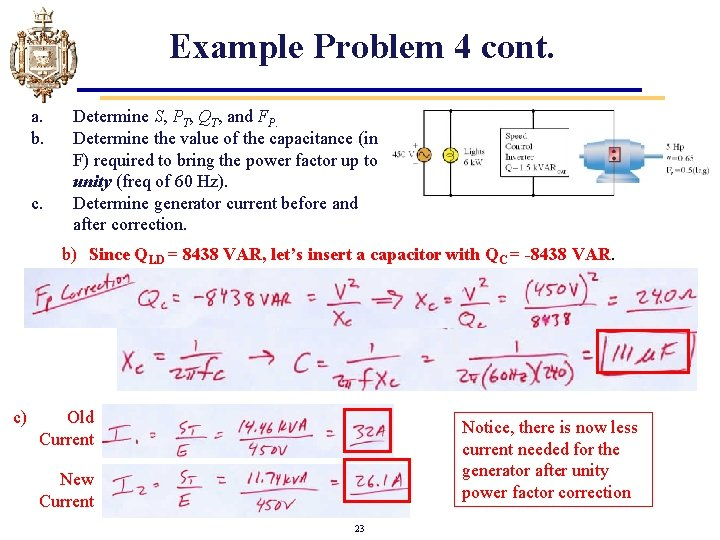Example Problem 4 cont. a. b. c. Determine S, PT, QT, and FP. Determine the value of the capacitance (in F) required to bring the power factor up to unity (freq of 60 Hz). Determine generator current before and after correction. b) Since QLD = 8438 VAR, let’s insert a capacitor with QC = -8438 VAR. c) Old Current Notice, there is now less current needed for the generator after unity power factor correction New Current 23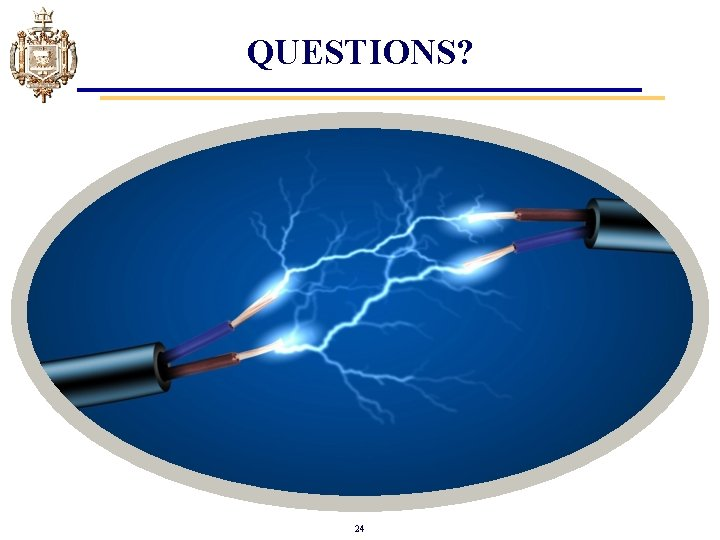QUESTIONS? 24